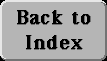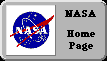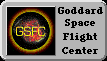# (M-1) Algebra--the basic ideas

Don't expect here a full-blown course on high-school algebra; it cannot be done, not in such limited space. These are just the bare bones, the basic ideas and rules of handling equations

In most calculations you try to find a number. For instance, the area of a rectangular plot of land 25 meters long and 40 meters wide (or yards, or feet) is

25 × 40 = 1000 square meters

Until the multiplication is carried out, we may represent the answer by some letter, usually x, and write

25 × 40 = x

One can then say "x stands for the unknown quantity". The fundamental idea of algebra is very simple:

The unknown quantity x is a number like any other. It may be added, subtracted, divided or multiplied in any way appropriate for ordinary numbers.

A mathematical relationship involving known numbers (like 25 or 40) and unknown ones (like x) is known as an equation. Often x is not given as cleanly as above, but is buried inside some complicated expression. To get a solution, one must replace the given equation (or equations) with others, containing the same information but cleaner in appearance. The final goal is to isolate the unknown, to make it stand apart ("isola" is island in Italian), to bring the equation to the above form, namely

x = (expression containing only known numbers)

Once that form is reached, the number which x represents can be quickly calculated.

For instance:

"What is the number which, if you double it, then add 5 and divide the sum by 3, you get 3?"

Call that number x. The information stated here in words can also be written down in equation form:

(2x + 5)/ 3 = 3

Parentheses here enclose quantities handled like a single number, and 2x means "2 times x". In algebra, symbols (or parentheses) standing next to each other are understood to be multiplied. If you stick to this rule, you will never be confused by the similarity between the letter x and the multiplication sign ×.

(computer programs, by the way, usually represent multiplication by *, placed a little lower than here.)
Now a second fundamental idea in algebra is:

If you have an equation and modify both its sides in exactly the same way, what you get is also a valid equation.

You may add, subtract, multiply or divide any number you wish; as long as it's done equally to both sides of the equality, the result is still valid. Also, the new equation still contains the same information as before. (But don't multiply both sides by 0 and get 0 = 0 ; the result is correct, but all your information has now vanished into thin air.)

For example, the equation given earlier:

(2x + 5)/ 3 = 3

Multiply both sides by 3:

(2x + 5) = 9

Subtract 5 from both sides:

2x = 9 - 5 = 4

Divide both sides by 2:

x = 4/ 2 = 2

and you have the result, x = 2. High school algebra contains a good deal more, but the above simple rules, plus the basic goal "isolate the unknown number," will get you a long way.

One last step is frequently skipped, but should not be. Just to make sure you haven't made a mistake along the way, take the original equation

(2x + 5)/ 3 = 3

replace in it the unknown quantity x by the value you have derived--in this case, by the number 2--and check whether the two sides are indeed equal. If they are, you can rest assured that your answer is correct.

Next Stop:   #M-2    Al-Khorezmi and the Dawn of AlgebraAuthor and curator: David P. Stern, u5dps@lepvax.gsfc.nasa.gov
Last updated 25 February 1999